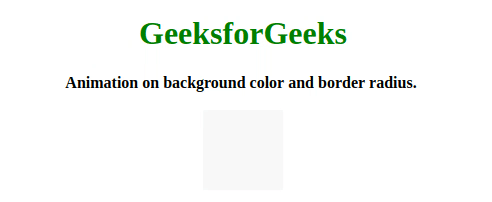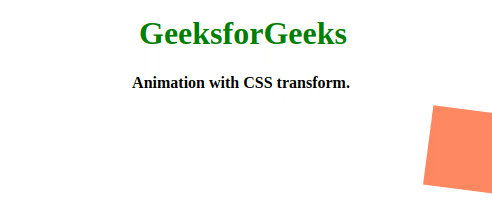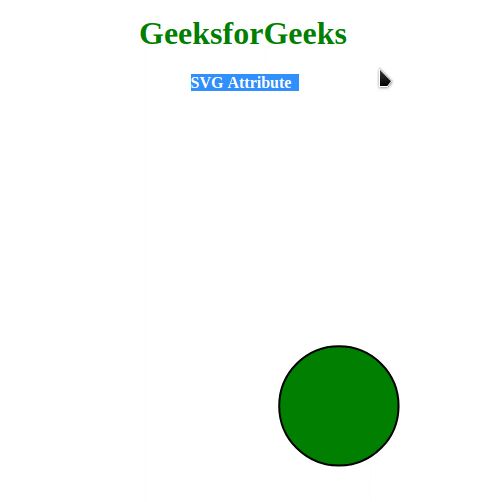Related Articles
Anime.js | Animation with different properties
• Last Updated : 02 Jun, 2020

Anime.js is a small, lightweight JavaScript library with a simple and small powerful API. It works with DOM element, CSS and JavaScript object. Animation with CSS properties  we can animate any CSS property. Below few of the examples are described with different CSS properties:

Example 1:  First we have to set a target  the div or element can be class or id, which we want to animate. The CSS background-color is one of the CSS property we are changing here from blue to #F75. The CSS border radius is another CSS property we are changing here. The we will use easing, easeInOutQuad mean just fading and showing, after that we will use the loop – true  to keep repeating the animation. You can animate any css property here we showed backgroundColor and borderRadius.

## html

 ```<``html``>``    ``<``head``>``        ``<``title``>animejs``        ``<``script` `src``=``"https://cdnjs.cloudflare.com/ajax/libs/animejs/3.2.0/anime.min.js"``>``       ````    ````    ``<``body``>``        ``<``center``>``            ``<``h1` `style``=``"color: green;"``>GeeksforGeeks``            ``<``b``>``                ``Animation on background ``                ``color and border radius.``            ````            ``<``br``><``br``>``            ``<``div` `style="background: blue; ``                        ``height: 80px;``                        ``width: 80px;">``            ````        ````        ``<``script``>``            ``let animation = anime({``                ``targets: "div",``                ``backgroundColor: "#F75",``                ``borderRadius: "50px",``                ``easing: "easeInOutQuad",``                ``loop: true,``            ``});``        ````    `````

Output:Example 2:  In this example, we will animate with CSS transform. First, we have to set a target  the div or element can be class or id, which we want to animate. The CSS translateX() function will set at 450 to move 450 into positive x-axis). We will use the CSS rotate() function to rotate – 720 degree. The CSS background-color is CSS property but not the part of CSS translate but we can use to change colour. The CSS direction property is set to alternate (move it back and fourth). Then the CSS duration is set for 2000 time for animation in ms) . after that we will use the loop – true  to keep repeating the animation.

## html

 ```<``html``>``    ``<``head``>``        ``<``title``>animejs``        ``<``script` `src``=``"https://cdnjs.cloudflare.com/ajax/libs/animejs/3.2.0/anime.min.js"``>``       ````    ````    ``<``body``>``        ``<``center``>``            ``<``h1` `style``=``"color: green;"``>GeeksforGeeks``            ``<``b``>``                ``Animation on background ``                ``color and border radius.``            ````            ``<``br``><``br``>``        ````            ``<``div` `style="background: blue; ``                               ``height: 80px;``                               ``width: 80px;">``            ````        ``<``script``>``            ``let animation = anime({``                ``targets: "div",``               ` `               ``// Move 250 into positive x-axis``                ``translateX: 450, ``               ` `               ``// Degree you want to rotate``                ``rotate: "720", ``                ``backgroundColor: "#f75",``                ``direction: "alternate",``                ``duration: "2000",``                ``loop: true,``            ``});``        ````    `````

Output:Example 3: Any Object property containing a numerical value can be animated. Here we just changed the value of object property and updated the dom and easing: linear is used to animate it linearly and you can change the round value to see what it is used for.

## html

 ```<``html``>``    ``<``head``>``        ``<``title``>animejs``        ``<``script` `src``=``"https://cdnjs.cloudflare.com/ajax/libs/animejs/3.2.0/anime.min.js"``>``        ````    ````    ``<``body``>``        ``<``div``>``        ``<``script``>``            ``var div = document.querySelector("div");`` ` `            ``var object = {``                ``prop1: 10,``                ``prop2: "0%",``            ``};`` ` `            ``let animation = anime({``                ``targets: object,``                ``prop1: 70,``                ``prop2: "96%",``                ``easing: "linear",``                ``round: 1,``                ``update: function () {``                    ``div.innerHTML = JSON.stringify(object);``                ``},``            ``});``        ````    `````

Output:Example 4: Here, values will contain from value – to value( Dom Attribute ). Such as value inside the input field (numeric)

## html

 ```<``html``>``    ``<``head``>``        ``<``title``>animejs``        ``<``script` `src``=``"https://cdnjs.cloudflare.com/ajax/libs/animejs/3.2.0/anime.min.js"``>``        ````    ````    ``<``body``>``        ``<``input` `style``=``"text-align: center;"` `type``=``"text"` `/>``        ``<``script``>``            ``let animation = anime({``                ``targets: "input",``                ``value: [0, 100],``                ``round: 1,``                ``easing: "easeInOutExpo",``            ``});``        ````    `````

Output:Example 5: In this example we will animate  SVG attribute. We created a circle of radius 50 centre at position 150, 150, then we applied anime on circle moved the centre to 200, 200 and made radius 60.

## html

 ```<``html``>``    ``<``head``>``        ``<``title``>animejs``        ``<``script` `src``=``"https://cdnjs.cloudflare.com/ajax/libs/animejs/3.2.0/anime.min.js"``>``        ````    ````    ``<``body``>``        ``<``center``>``            ``<``h1` `style``=``"color: green;"``>GeeksforGeeks``            ``<``b``>``                ``SVG Attribute``            ````            ``<``br``><``br``>``            ``<``svg` `height``=``"400"` `width``=``"400"``>``                ``<``circle` `cx``=``"50"` `cy``=``"50"` `r``=``"40"` `                        ``stroke``=``"black"` `stroke-width``=``"2"` `                        ``fill``=``"green"``/>``            ````        ````        ``<``script``>``            ``let animation = anime({``                ``targets: ["circle"],``                ``cx: "300",``                ``cy: "300",``                ``r: "60",``                ``baseFrequency: 0,``                ``scale: 1,``                ``loop: true,``                ``direction: "alternate",``                ``easing: "easeInOutExpo",``            ``});``        ````    `````

Output:My Personal Notes arrow_drop_up